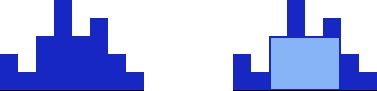# Pattern - Largest Rectangle in a Histogram

Time Limit : 1 sec, Memory Limit : 262144 KB
Japanese version is here

# Largest Rectangle in a Histogram

A histogram is made of a number of contiguous bars, which have same width.

For a given histogram with $N$ bars which have a width of 1 and a height of $h_i$ = $h_1, h_2, ... , h_N$ respectively, find the area of the largest rectangular area.

## Constraints

• $1 \leq N \leq 10^5$
• $0 \leq h_i \leq 10^9$

## Input

The input is given in the following format.

$N$
$h_1$ $h_2$ ... $h_N$

## Output

Print the area of the largest rectangle.

## Sample Input 1

8
2 1 3 5 3 4 2 1


## Sample Output 1

12## Sample Input 2

3
2 0 1


## Sample Output 2

2